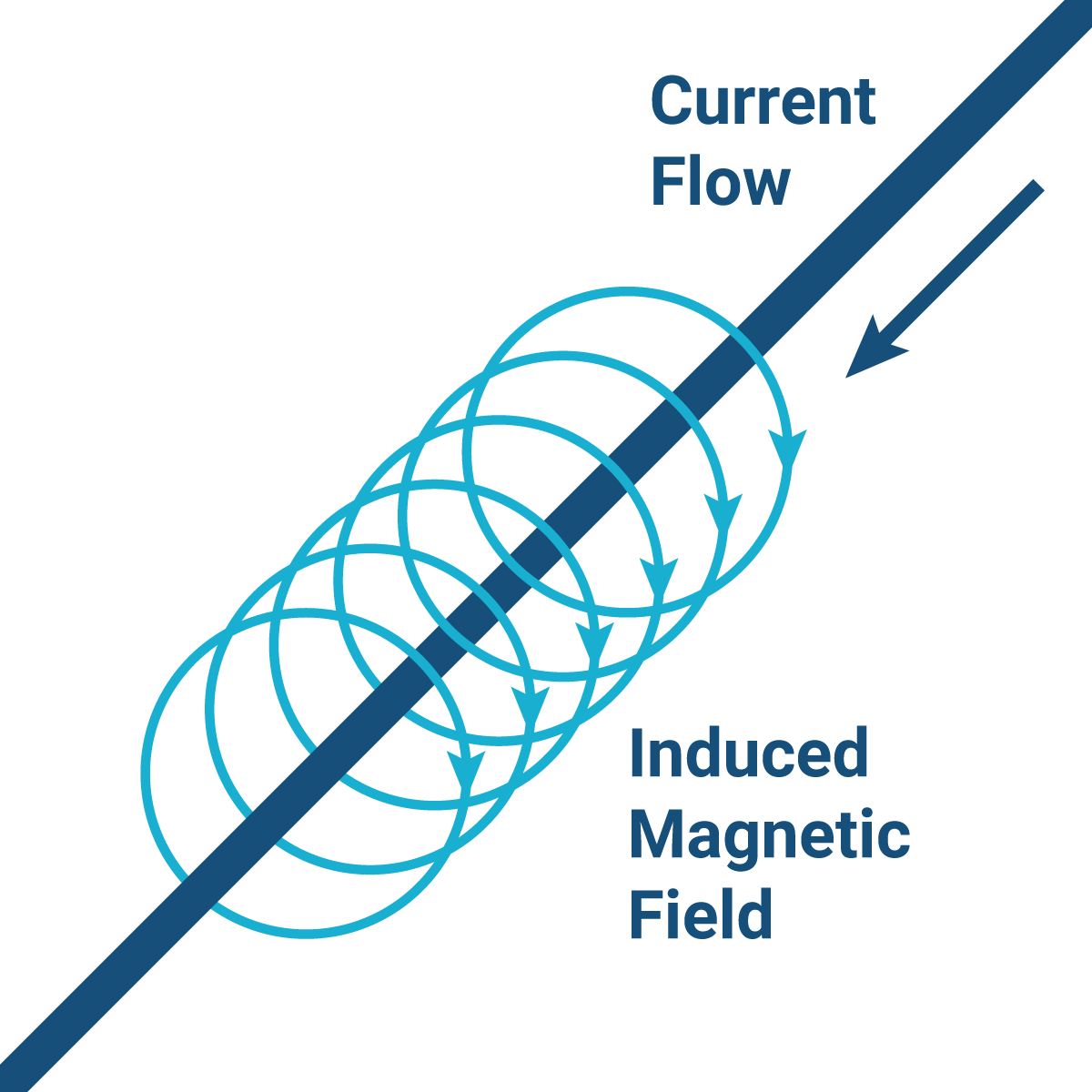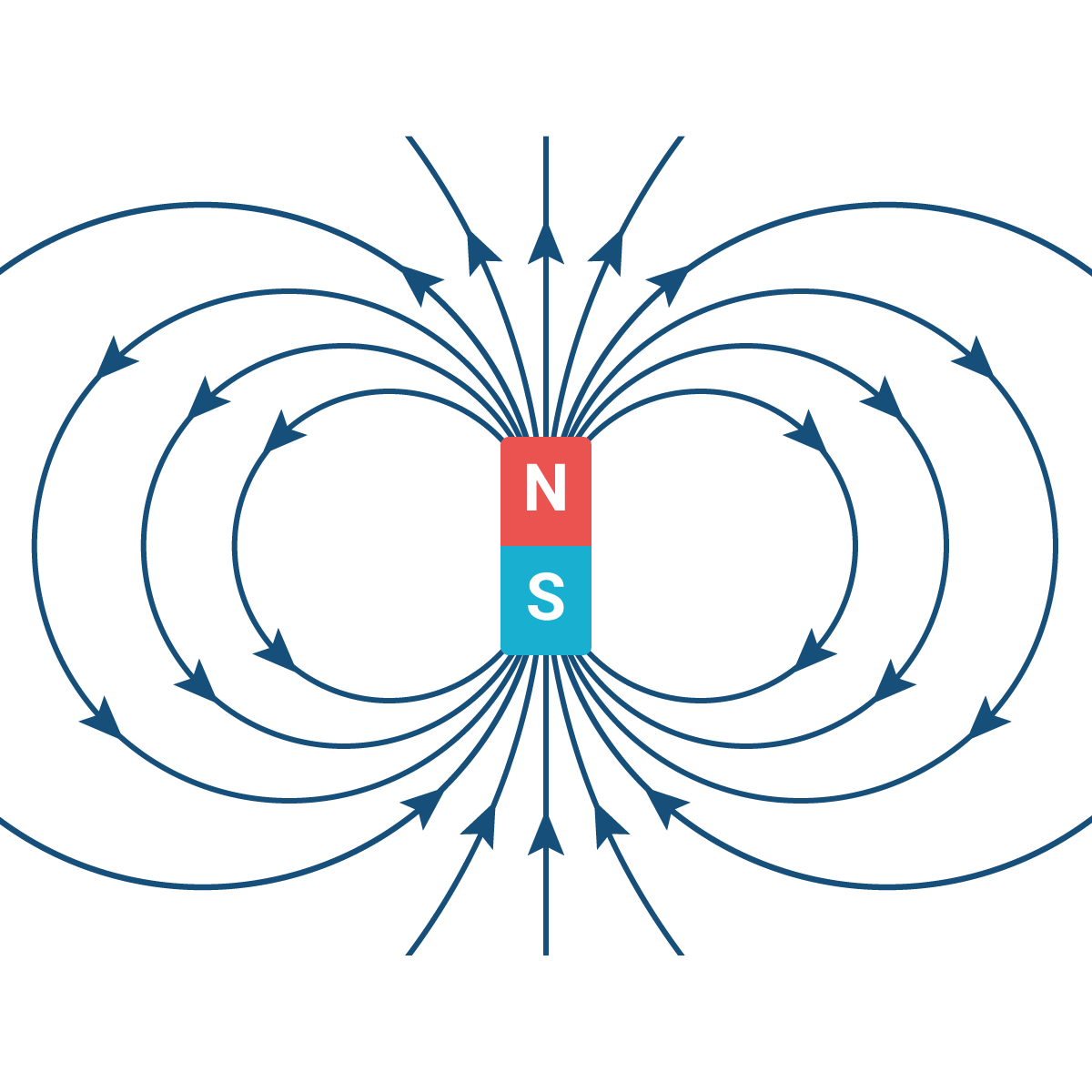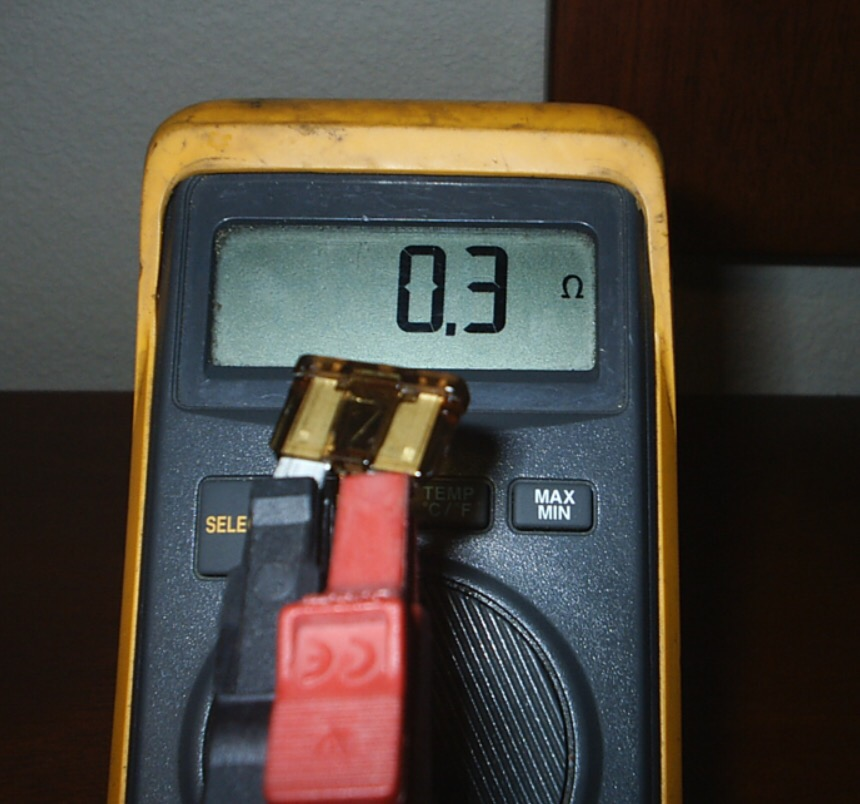## What is Inductive Reactance?Ohm’s Law is pretty straightforward; you multiply ohms by amps to get the voltage. Using variable E to represent voltage, variable I for amps, and variable R for ohms, the equation for Ohm’s Law looks like this:

E = I × R

You can figure out the number of amps in a system using basic algebra to turn this multiplication equation into a division one. Divide both sides by R to isolate the amperage (E/R = I). From there, you’d take the voltage reading and divide it by your ohm reading. Your equation should yield the amperage.

However, if you’ve tried doing that and then comparing your answer to the actual amperage measurement, you’ll know that there’s a lot less current than the equation would lead you to believe. Ohm’s Law appears to be inaccurate most of the time, and it’s a bit frustrating because there’s such an emphasis on it in electrical education, but it doesn’t seem to work in the field. Why do we even learn about it in the first place?

The truth is that Ohm’s Law is still valid and works just fine. It’s merely impractical for many of the alternating current (AC) components we work on. That’s because the ohms we measure don’t account for all resistance types that make up total impedance. Inductive reactance is one of those types of impedance, and our multimeters and ohmmeters can’t pick it up. It also happens to be a byproduct of the inductive loads we regularly use.##### Loads

Maybe I’ve gotten a bit ahead of myself by tacking “inductive” onto words without explaining them. So, what is a load, anyway?

Simply put, a load is a component that does something in an electrical circuit. For example, a lightbulb is a load because it lights up when it receives power. In terms of the work we do, motors and transformers are loads that we regularly use.

There’s quite a difference between lightbulbs and motors or transformers. They each belong to different load categories; lightbulbs are resistive loads, while motors and transformers are inductive loads. Resistive loads have a heating component (toasters, oven coils, and electric heaters are also resistive loads), and inductive loads have an electromagnetic element.

We see plenty of inductive AC loads in the work we do. (AC refers to alternating current. Inductors don’t have a significant effect on DC circuits.) Inductive loads facilitate magnetism and (usually) mechanical movement.

Transformers are the exception to the movement rule. Transformers only transfer electric energy via electromagnetism and don’t have any moving parts. Still, the point stands that magnetism is the core trait of inductive loads.##### Electromagnetism

I don’t want to dwell on electromagnetism for too long. Still, I think we should have a solid grasp of its fundamentals before we discuss inductive reactance.

When current travels through a wire, it will make a small magnetic field. It stands to reason that a coiled wire over a small area would create a larger, stronger magnetic field. After all, the current runs through the wire several times in the same small space.

The magnetic field expands as the current runs through the coil, and electrical energy accumulates as magnetic energy when the field is at its maximum size. When the current stops flowing, the field shrinks until it disappears entirely, returning all the stored energy to electric energy. It takes a bit of time to store and release the power, so you’ll always see a lag in the current.

##### Magnetism vs. heat

As we just explained, inductive loads hold their energy in magnetic fields. This energy storage method is why resistive loads heat up quickly, but inductive loads do not.

The magnetic field’s energy storage impedes the current. The current doesn’t travel from point A to point B in the circuit without experiencing that delay. The delay reduces the total power delivered, so inductive loads don’t heat up to the same degree as resistive loads.

On the other hand, voltage and current peak simultaneously in resistive loads, which allows all the power in the circuit to be delivered. Resistive loads heat up much more quickly because their circuits don’t have the same delay as inductive load circuits. That’s why solenoids, relay coils, and motors don’t act as heaters that overload constantly.

##### Reactance and impedance

Reactance is a component’s opposition to the current flow, just like the lag we talked about in the previous sections. As this description suggests, reactance is a form of resistance.

Reactance is a type of resistance called impedance. (Remember when I said that the energy storage delay impedes the current?) As such, inductive reactance is impedance from inductive loads. Although lightbulbs and other resistive loads present some form of resistance while operating, the resistance from inductive loads is significantly higher.

The total impedance is a combination of reactance and resistive ohms, so they both make up the total number of ohms.

Like other sources of resistance, we measure reactance in ohms. However, as I said earlier, you can only use your multimeter or ohmmeter to measure resistive ohms. An ohmmeter can't measure reactance, so there's no way you can measure inductive reactance beforehand. As a result, Ohm’s Law can't be used to find current by measuring ohms in most of what we do.

The design of electrical components dictates the resistance and impedance within them. The wires’ winding affects the behavior of inductive reactance, ohmic resistance, and current, as you’ll read shortly.

##### Inductive reactance and current

You may have noticed that motors draw higher current upon startup. Many people call this the inrush current, which will typically be 4-6 times higher than the standard running current.

The current is strongest at the start because it takes a little bit of time for the impedance to push back against the current resistance. That usually happens after the motor starts spinning. However, once the inductive reactance has established itself, it strongly resists the current and reduces the amperage as a result.

If you use Ohm’s Law to find the amperage and yield a number that’s much higher than your ohmmeter’s reading, that’s because you haven’t accounted for the effect that inductive reactance has on current. Again, inductive reactance won’t show up on ohmage readings, but it still impedes the amperage and results in a much lower amperage reading than expected.

##### Winding: inductive reactance and transformers

We’ve already established that transformers are the odd ones out because they lack moving parts. Instead, transformers transfer electrical energy from one circuit to another via electromagnetic induction.

Transformers have two sets of windings: primary and secondary. The primary winding connects directly to the AC supply, and the secondary winding connects to the load (output terminal). A magnetic core binds the primary and secondary winding.

When a transformer has no load on the secondary winding, it draws almost no current on the primary winding. That’s because the impedance on the secondary winding is extremely high, and it becomes a near-perfect inductor when there is no load.

Did we really go through all this information just to prove that Ohm’s Law is not a sham? Absolutely. Our ohmmeters and multimeters only give us part of the picture, and it’s unfair (and inaccurate) to judge the validity of Ohm’s Law with our limited measurements.

As you can see, the electrical world is complicated, and there’s a lot more to resistance than the ohms we can measure with our devices. Just remember that the amps don’t magically disappear; they get impeded by inductive reactance.

## Parallel Circuit ResistanceIn a Series circuit (loads connected in a row end to end) it's easy to calculate total circuit resistance because you simply add up all the resistances and you have the total.

In a Parallel circuit the voltage is the same across all the loads, the amperage is simply added up but the resistance is a bit more tricky.

It gets tricky to imagine because the total circuit resistance of parallel loads goes down the more loads you add.

For example, if you have one light bulb connected to a power source, the total resistance of the circuit is just the resistance of the bulb.

Add in another bulb in PARALLEL and the resistance of the circuit goes DOWN

When you are calculating the total resistance of a parallel circuit you take each individual resistance and divide it into (not by) one. You then add up all the resistances that were divided into one and divide that sum into one. The formula looks like this for the diagram at the top of the article.

1÷Rt (total resistance)= 1÷R1 + 1÷R2 + 1÷R3

For this particular application as shown above it would be.

1÷Rt(total resistance)=1÷120 + 1÷45 + 1÷360

So 1 ÷ 120 = .0083 + 1 ÷ 45 = .022 + 1 ÷ 360 = .0028

Then we add them all up

.0083 + .022 + .0028 = .0331

Then to find the total you divide one by the total

1 ÷ .0331 = 30.21 Ohms total

As you will notice, 30.21 Ohms is less than the lowest resistance in the circuit. This makes sense when you think about ohms law.

The lower the resistance the higher the amps. Adding in additional parallel loads INCREASES the amperage in a circuit, and we see this ever day when we notice that compressor amps and condenser fan amps added together equals total condenser amps.

So it stands to reason if lower resistance equals higher amps and adding in more parallel loads increases the amps, then adding in more parallel loads reduces the resistance.

Another myth this busts is the idea that electricity ONLY takes the path of least resistance. Electricity actually takes all paths between positive and negative charges and every additional path (parallel circuit) just decreases the resistance between the two points of potential difference. This increases the total circuit amperage, which is why when you try to run two hair dryers on one 15a circuit the breaker trips. Two hair dryers in parallel = lower  total circuit resistance = higher amps.

Not that I would use two hair dryers….. maybe that's why I'm almost bald.

— Bryan

## Ohm myOne of the most common mistakes I hear techs make is confusing Zero ohms with infinite ohms. The fuse above is showing near zero Ohms which means a good electrical path with very little resistance.

If there is a perfect path it would have zero ohms (which isn't actually possible unless you happen to be testing a superconductor).

If there is no path, the circuit has infinite ohms. This would be shown as Open or OL or something similar.

Often when I ask what ohm reading a tech is getting they will say “none”… None could easily mean zero or infinite so it's important to clarify.

Once again.

Zero ohms = shorted / closed / directly connected

Infinite ohms = open/ no path

Try to remember to say either Infinite or zero instead of  “no ohms” or “none” to avoid confusion

— Bryan

## Ohms / Continuity BasicsSome quick basics –

An Ohmmeter is used to measure the resistance to electrical flow between two points. The Ohmmeter is most commonly used to check continuity. Continuity is not a “measurement” as much as it is a yes / no statement. To say there is continuity is to say that there is a good electrical path between two points. To say there is no continuity means there is not a good electrical path.

In other words, continuity means low or zero ohms and no continuity means very high or infinite ohms. Don't get the terms zero ohms and infinite ohms confused, they mean opposite things.

This type of testing is commonly used to check fuses, Trace wires, check for short and open circuits Etc… Resistance readings are necessary for identifying motor terminals, and checking for a breakdown in insulation. An Ohmmeter continuity can be used to identify normally open, and closed terminals on a relay. Simply place the leads of the meter across the relay points, if there is continuity the relay is normally closed. Now apply power to the magnetic coil of the relay, the contacts that were closed should now open, or vice versa. An Ohmmeter can be used to identify a single wire in a bundle. Go to the opposite end of the wire and expose two separate wires in one sheath. Twist the two wires together and list the colors. Go back to the other end and check for continuity between all wires of that color.

Once you find two wires with continuity, you have found the correct wire. If you suspect that a particular wire is shorted to another wire, simply disconnect both wires on each end and check for continuity between the two wires. If continuity is read between the wires you have found a short.

These are only a few examples of ways to utilize an Ohmmeter.  Remember an Ohmmeter should only be used in un-energized circuits, Otherwise the meter could be damaged.

— Bryan

loading

### To continue you need to agree to our terms.English